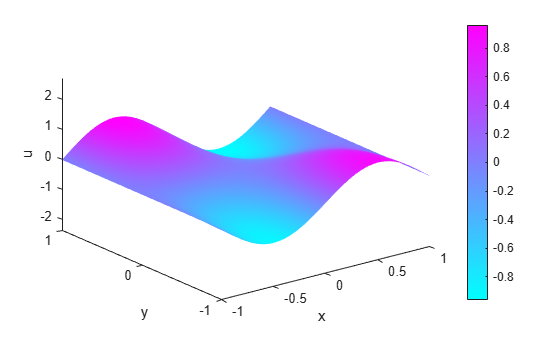# Wave Equation on Square Domain

This example shows how to solve the wave equation using the `solvepde` function.

The standard second-order wave equation is

`$\frac{{\partial }^{2}u}{\partial {t}^{2}}-\nabla \cdot \nabla u=0.$`

To express this in toolbox form, note that the `solvepde` function solves problems of the form

`$m\frac{{\partial }^{2}u}{\partial {t}^{2}}-\nabla \cdot \left(c\nabla u\right)+au=f.$`

So the standard wave equation has coefficients $m=1$, $c=1$, $a=0$, and $f=0$.

```c = 1; a = 0; f = 0; m = 1;```

Solve the problem on a square domain. The `squareg` function describes this geometry. Create a `model` object and include the geometry. Plot the geometry and view the edge labels.

```numberOfPDE = 1; model = createpde(numberOfPDE); geometryFromEdges(model,@squareg); pdegplot(model,'EdgeLabels','on'); ylim([-1.1 1.1]); axis equal title 'Geometry With Edge Labels Displayed'; xlabel x ylabel y```Specify PDE coefficients.

`specifyCoefficients(model,'m',m,'d',0,'c',c,'a',a,'f',f);`

Set zero Dirichlet boundary conditions on the left (edge 4) and right (edge 2) and zero Neumann boundary conditions on the top (edge 1) and bottom (edge 3).

```applyBoundaryCondition(model,'dirichlet','Edge',[2,4],'u',0); applyBoundaryCondition(model,'neumann','Edge',([1 3]),'g',0);```

Create and view a finite element mesh for the problem.

```generateMesh(model); figure pdemesh(model); ylim([-1.1 1.1]); axis equal xlabel x ylabel y```Set the following initial conditions:

• $u\left(x,0\right)=\mathrm{arctan}\left(\mathrm{cos}\left(\frac{\pi x}{2}\right)\right)$.

• ${\frac{\partial u}{\partial t}|}_{t=0}=3\mathrm{sin}\left(\pi x\right)\mathrm{exp}\left(\mathrm{sin}\left(\frac{\pi y}{2}\right)\right)$.

```u0 = @(location) atan(cos(pi/2*location.x)); ut0 = @(location) 3*sin(pi*location.x).*exp(sin(pi/2*location.y)); setInitialConditions(model,u0,ut0);```

This choice avoids putting energy into the higher vibration modes and permits a reasonable time step size.

Specify the solution times as 31 equally-spaced points in time from 0 to 5.

```n = 31; tlist = linspace(0,5,n);```

Set the `SolverOptions.ReportStatistics` of `model` to `'on'`.

```model.SolverOptions.ReportStatistics ='on'; result = solvepde(model,tlist);```
```459 successful steps 39 failed attempts 998 function evaluations 1 partial derivatives 115 LU decompositions 997 solutions of linear systems ```
`u = result.NodalSolution;`

Create an animation to visualize the solution for all time steps. Keep a fixed vertical scale by first calculating the maximum and minimum values of `u` over all times, and scale all plots to use those $z$-axis limits.

```figure umax = max(max(u)); umin = min(min(u)); for i = 1:n pdeplot(model,'XYData',u(:,i),'ZData',u(:,i),'ZStyle','continuous',... 'Mesh','off','XYGrid','on','ColorBar','off'); axis([-1 1 -1 1 umin umax]); caxis([umin umax]); xlabel x ylabel y zlabel u M(i) = getframe; end```To play the animation, use the `movie(M)` command.

## SupportGet trial now